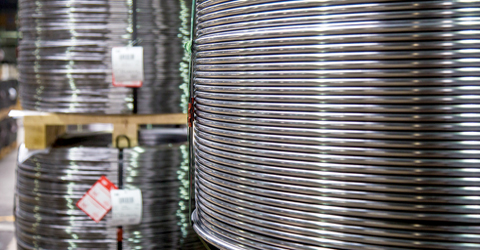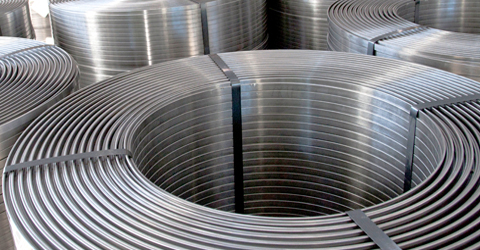•••••••# Drawn wire, cold rolled flat wire and copper coated wire

## Presentation of SIAT

SIAT SpA is one of the leading producers in Europé of drawn wire, cold rolled flat wire and copper coated wire. Material could be different types of low carbon steel from own steel melting. Company was founded in the 1970’s and is a part of Pittini Group, who produces steel with an annual capacity of 3 million tons. Production capacity of SIAT is 80 000 tons/year of cold rolled flats and 40 000 ton/year of drawn wire and they are certified according to ISO 9001:2008.

The company is located in Udine in Northeast of Italy, close to the Austrian border with good road- and railway connections.

## Product range

The product range is drawn wire, cold rolled flat wire and copper coated wire:

• Drawn wire Ø1.6 - 14 mm
• Cold rolled flat wire 7x2 to 30x4 mm, se table below
• Copper coated wire Ø1.5-5 mm

Drawn wire and cold rolled flat wire is delivered in following steel grades: C4D, C7D, D9D according to EN ISO 16120-2 (drawn wire) respectively EN 10139 (cold rolled flat wire).

## Technical details

For further information about produced dimensions of cold rolled flat wire, see table below.

For more detailed information about packing, coil weight and coil sizes, see respective product sheet.

Thickness (mm)
789101112131415161718192021222324252627282930
Width
(mm)
1.5
X
X
X
X
X
X
X
X
X
1.6
X
X
X
X
X
X
X
X
X
1.7
X
X
X
X
X
X
X
X
X
X
X
X
X
X
X
1.8
X
X
X
X
X
X
X
X
X
X
X
X
X
X
X
X
X
1.9
X
X
X
X
X
X
X
X
X
X
X
X
X
X
X
X
X
2
X
X
X
X
X
X
X
X
X
X
X
X
X
X
X
X
X
X
X
3
X
X
X
X
X
X
X
X
X
X
X
X
X
X
X
X
X
X
X
X
X
X
X
X
4
X
X
X
X
X
X
X
X
X
X
X
X
X
X
X
X
X
X
X
X
X
X
X
X
5
X
X
X
X
X
X
X
X
X
X
X
X
X
X
X
X
X
X
X
X
X
X
X
X
6
X
X
X
X
X
X
X
X
X
X
X
X
X
X
X
X
X
X
7
X
X
X
X
X
X
X
X
X
X
X
X
X
X
X
X
X
8
X
X
X
X
X
X
X
X
X
X
X
X
X
X
X
9
X
X
X
X
X
X
X
X
X
X
X
X
X
10
X
X
X
X
X
X
X
X
X
X
X
11
X
X
X
X
X
X
X
X
X
12
X
X
X
X
X
X
X